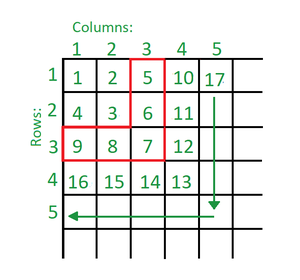GeeksforGeeks App
Open AppBrowser
Continue

# Find the position of given element N in infinite spiral matrix starting from top-left

Given a number N and an infinite 2D matrix, which is to be filled using the algorithm given below, the task is to find the coordinates of the given element present in the matrix. The algorithm is as follows:

• The leftmost and topmost cell of the matrix is filled with 1. Then, all the cells are filled with consecutive numbers starting from 2
• The leftmost non-filled cell in the first row is filled. Then, while the left neighbor of the last filled cell is filled, the cells below it are filled next, until the last filled cell has a non-filled left neighbor
• Next, the cells are filled from right to the left until the first column. Then, again the leftmost non-filled cell in the first row is filled
• The above two steps are repeated infinitelyExamples:

Input: N = 12
Output: 3 4
Explanation: 12 lies in the cell with row as 3 and column as 4

Input: N = 10549857
Output: 353 3249
Explanation: 10549857 lies in the cell with row as 353 and column as 3249

Approach: Following observations can be made:

• In the above image that the red highlighted portion or ‘inverted L’ formed by the 3rd row and 3rd column consists of all numbers greater than 4 and smaller than 10. Similarly, the ‘inverted L’ formed by the 4th row and 4th column consists of numbers greater than 9 and smaller than 17
• In other words, numbers present are excluding the current perfect square till including the next perfect square
• To find the ‘inverted L’ in which the number lies, find the ceil of the square root of the number
• The difference between the square of ‘inverted L’ and the given number is calculated, say d
• If l is the ‘inverted L’ in which the number lies and n denotes the given number, then:

d = l^2 – N

• If this difference d is less than n, then the row r and column c of n is given by:

r = l
c = d + l

• If this difference d is greater than or equal to n, then the row r and column c of n is given by:

r = 2l – d – 1
c = l

Below is the implementation of the above approach:

## C++

 `// C++ Implementation for the above approach` `#include ``using` `namespace` `std;` `// Function to find the coordinates of n``void` `findCoordinates(``int` `n)``{``    ``// Store the row and column of``    ``// n respectively``    ``int` `r, c;` `    ``// Stores the inverted L``    ``// in which n lies``    ``int` `l = ``ceil``(``sqrt``(n));` `    ``// Stores the difference between``    ``// square of l and n``    ``int` `d = (l * l) - n;` `    ``// If d is less than l``    ``if` `(d < l) {``        ``r = l;``        ``c = d + 1;``    ``}``    ``else` `{``        ``c = l;``        ``r = (2 * l) - d - 1;``    ``}` `    ``cout << r << ``" "` `<< c;``}` `// Driver code``int` `main()``{``    ``// Given n``    ``int` `N = 10549857;` `    ``// Function call``    ``findCoordinates(N);` `    ``return` `0;``}`

## Java

 `// Java Implementation for the above approach` `public` `class` `GFG {``    ` `// Function to find the coordinates of n``static` `void` `findCoordinates(``int` `n)``{``  ` `    ``// Store the row and column of``    ``// n respectively``    ``int` `r, c;` `    ``// Stores the inverted L``    ``// in which n lies``    ``int` `l = (``int``)Math.ceil(Math.sqrt(n));` `    ``// Stores the difference between``    ``// square of l and n``    ``int` `d = (l * l) - n;` `    ``// If d is less than l``    ``if` `(d < l) {``        ``r = l;``        ``c = d + ``1``;``    ``}``    ``else` `{``        ``c = l;``        ``r = (``2` `* l) - d - ``1``;``    ``}` `    ``System.out.print(r + ``" "` `+ c);``}` `    ``// Driver code``    ``public` `static` `void` `main (String[] args) {``        ``// Given n``        ``int` `N = ``10549857``;``    ` `        ``// Function call``        ``findCoordinates(N);` `    ``}``}` `// This code is contributed by AnkThon`

## Python3

 `# Python Program to implement``# the above approach``import` `math` `# Function to find the coordinates of n``def` `findCoordinates(n):``  ` `    ``# Store the row and column of``    ``# n respectively``    ``#let r, c` `    ``# Stores the inverted L``    ``# in which n lies``    ``l ``=` `math.ceil(math.sqrt(n))` `    ``# Stores the difference between``    ``# square of l and n``    ``d ``=` `(l ``*` `l) ``-` `n` `    ``# If d is less than l``    ``if` `(d < l):``        ``r ``=` `l``        ``c ``=` `d ``+` `1``    ``else``:``        ``c ``=` `l``        ``r ``=` `(``2` `*` `l) ``-` `d ``-` `1` `    ``print``(f``"{r} {c}"``)` `# Driver code` `# Given n``N ``=` `10549857` `# Function call``findCoordinates(N)` `# This code is contributed by Saurabh Jaiswal`

## C#

 `// C# Implementation for the above approach``using` `System;``public` `class` `GFG {``    ` `// Function to find the coordinates of n``static` `void` `findCoordinates(``int` `n)``{``  ` `    ``// Store the row and column of``    ``// n respectively``    ``int` `r, c;` `    ``// Stores the inverted L``    ``// in which n lies``    ``int` `l = (``int``)Math.Ceiling(Math.Sqrt(n));` `    ``// Stores the difference between``    ``// square of l and n``    ``int` `d = (l * l) - n;` `    ``// If d is less than l``    ``if` `(d < l) {``        ``r = l;``        ``c = d + 1;``    ``}``    ``else` `{``        ``c = l;``        ``r = (2 * l) - d - 1;``    ``}` `    ``Console.Write(r + ``" "` `+ c);``}` `    ``// Driver code``    ``public` `static` `void` `Main (``string``[] args) {``        ``// Given n``        ``int` `N = 10549857;``    ` `        ``// Function call``        ``findCoordinates(N);` `    ``}``}` `// This code is contributed by shivanisinghss2110`

## Javascript

 ``

Output

`353 3249`

Time Complexity: O(log(n)) because inbuilt sqrt function has been used
Auxiliary Space: O(1)

My Personal Notes arrow_drop_up